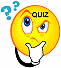Professor Freedman's
Prealgebra Homework Schedule
Fall 2020

Text: Prealgebra by Miller, O'Neill & Hyde, 3rd Edition, McGrawHill, Inc.,2020

Assignments

WEEK of 9/2

Homework Assigned

Watch the following videos:

Introduction to Algebra -Exponents, Absolute Values & Order of Operations

Addition & Subtraction of Signed Numbers

Complete in Aleks.com Assignments #1 & 2

Now complete quiz #1 in Aleks.comQUIZ #1 in Aleks.com on the first 2 videos

Homework Tips (Week 1)

WEEK of 9/7

Homework Assigned

Watch the following videos:

Multiplication & Division of Signed Numbers

Order of Operations on Signed Numbers

Now complete Assignment #3 and quiz #2 in Aleks.comQUIZ #2 in Aleks.com on the Multiplication & Division of Signed Numbers

Watch the following videos:

Evaluating an Algebraic Expression

Commutative, Associative & Distributive Properties

Combining Like Terms

Now complete assignment #4 in Aleks.com

Homework Tips (Week 2)

WEEK of 9/14

 Review Evaluating Algebraic Expression, Properties, & Combining Like Terms & Begin Solving Equations(Part 1) Homework Assigned: Watch the following videos: Evaluating an Algebraic Expression Commutative, Associative & Distributive Properties Combining Like Terms Solving Linear Equations (Part 1) Complete Assignment #5 (Equations Part 1) andQUIZ #3 in Aleks.com

WEEK of 9/21

Solving Linear Equations with Multiple Steps (Part 2)
Complete Assignment # 6 in Aleks.comQUIZ #4 in Aleks.com

WEEK of 9/28

MAJOR TEST in ALEKS.com on Chapters,1,2,3

PRACTICE TEST

WEEK of 10/5

Homework Assigned:

Watch the following videos:

Proper & Improper Fractions & Mixed Numbers

Converting Mixed Numbers to Improper Fractions

Simplifying Fractions

Multiplying Fractions

Dividing Fractions

In Aleks.com Complete Assignment #7 (Proper & Improper Fractions), Assignmnet #8 (Simplifying Fractions), Assignment #9 ( Multiplication & Division of Fractions)QUIZ #5 in Aleks.com

WEEK of 10/12

Homework Assigned:

Watch the following videos:

Writing Equivalent Fractions

Ordering Fractions

Adding & Subtracting Fractions with Same Denominator

Adding & Subtracting Fractions with Different Denominators

Multiplication of Mixed Numbers

Division of Mixed Numbers

In Aleks.com Complete Assignment #10 (Equivalent Fraction and Ordering Fractions) , Assignment #11 (Add and Subtract Fractions), Assignment #12 ( Multiplication & Division of Mixed Numbers), Assignment #13 (Add Mixed Numbers)

WEEK of 10/19

Homework Assigned:

Watch the following videos:

Subtraction of Mixed Numbers

Order of Operations with Mixed Numbers

Rounding Decimals

Ordering Decimals

In Aleks.com Complete Assignment #14 (Subtraction of Mixed Numbers with Borrowing) , Assignment #15 (Order of Operations with Fractions), Assignment 16 (Decimal Notation & Rounding), Assignment #17 (Practice Test)

STUDY FOR MIDTERM EXAM - DO PRACTICE TEST Assignment #17 (Practice Test - Due 10/25)

WEEK of 10/26

STUDY FOR MIDTERM EXAM

MIDTERM EXAM DUE 11/1

WEEK of 11/2

Homework Assigned:

Watch the following videos:

Decimals - Multiplication & Division by Powers of Ten

In Aleks.com Complete Assignment #18 (Multiplying and Dividing by Powers of 10 and Rounding Quotients) , Assignment #19 (Writing Fractions to Decimals and Decimals to Fractions), Assignment 20 (Mean, Median, Mode,
Assignment #21 (Ratio and Rate)

WEEK of 11/9